Learning Library

# Veggie Math Mash: Two-Digit Subtraction

Make math palatable with these food-themed puzzles. With all of those veggies featured you'll be giving your kid some essential math practice and encouraging healthy eating habits at the same time.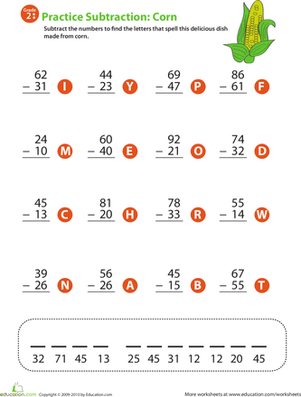### 2-Digit Subtraction

Give your child's math skills a boost with this 2nd grade subtraction worksheet that includes two-digit problems and a word puzzle at the end!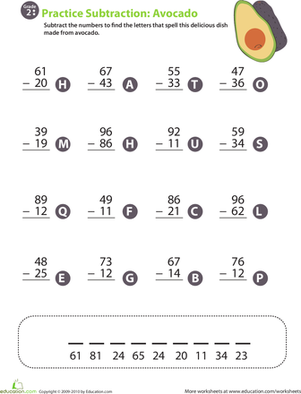### Veggie Math Mash: Two-Digit Subtraction 2

Give your child valuable at-home math practice with this 2nd grade worksheet that includes two-digit subtraction problems and a word puzzle at the end.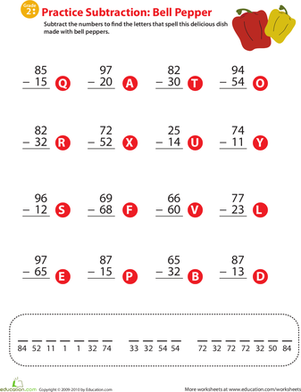### Veggie Math Mash: Two-Digit Subtraction 3

Satisfy your child's craving for math with this veggie-themed 2nd grade worksheet that offers two-digit subtraction problems and a word puzzle at the end.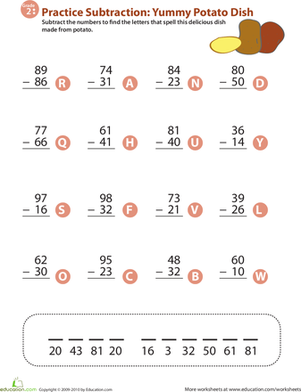### Veggie Math Mash: Two-Digit Subtraction 4

Offering 16 two-digit subtraction problems and a word puzzle at the end, this 2nd grade math worksheet provides a healthy dose of mental nutrition.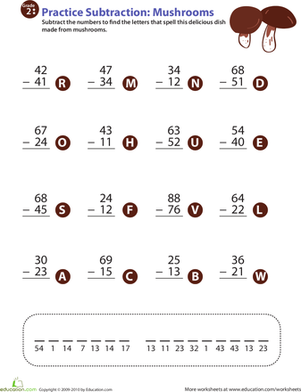### Veggie Math Mash: Two-Digit Subtraction 5

Improve your second grader's subtraction facts fluency with this mushroom-themed math worksheet that ends with a surprise: a word puzzle!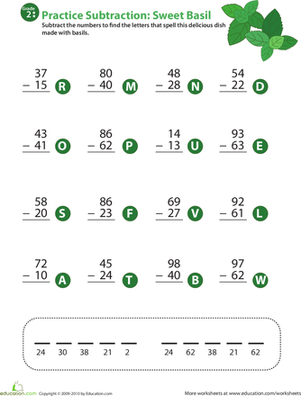### Veggie Math Mash: Two-Digit Subtraction 6

Help your second grader learn two-digit subtraction with this math worksheet that offers 16 subtraction problems and a fun word puzzle at the end.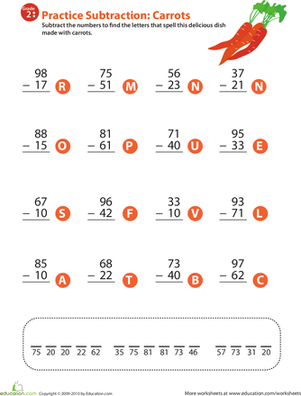### Veggie Math Mash: Two-Digit Subtraction 7

Carrots add fun and interest to this 2nd grade math worksheet that helps your child learn his subtraction facts and hone his mental math ability.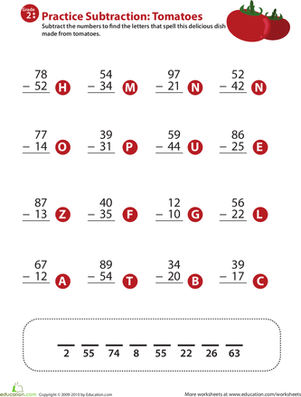### Veggie Math Mash: Two-Digit Subtraction 8

To help improve your child's subtraction facts fluency, download this cheery 2nd grade worksheet that offers 16 subtraction problems and a fun tomato theme.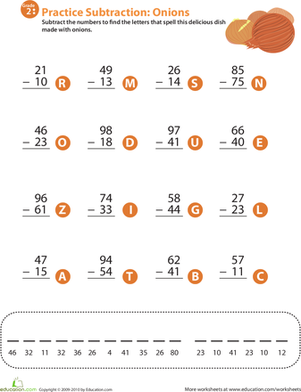### Veggie Math Mash: Two-Digit Subtraction 9

This 2nd grade math worksheet makes subtraction fun. Children complete the two-digit problems, then use their answers to solve a word puzzle.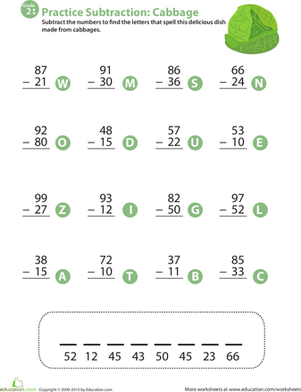### Veggie Math Mash: Two-Digit Subtraction 10

Explore the mathematical side of cabbage in this 2nd grade worksheet that combines two-digit subtraction practice with a cabbage-themed word puzzle.

Create new collection

0

### New Collection>

0 items

What could we do to improve Education.com?